## Network Theory (Part 7)

#### Jacob Biamonte

This post is part of a series on what John and I like to call Petri net field theory. Stochastic Petri nets can be used to model everything from vending machines to chemical reactions. Chemists have proven some powerful theorems about when these systems have equilibrium states. We're trying to bind these old ideas into our fancy framework, in hopes that quantum field theory techniques could also be useful in this deep subject. We'll describe the general theory later; today we'll do an example from population biology.

Those of you following this series should know that I'm the calculation bunny for this project, with John playing the role of the wolf. If I don't work quickly, drawing diagrams and trying to keep up with John's space-bending quasar of information, I'll be eaten alive! It's no joke, so please try to respond and pretend to enjoy anything you read here. This will keep me alive for longer. If I did not take notes during our meetings, lots of this stuff would have never made it here, so hope you enjoy.

### Amoeba reproduction and competition

Here's a stochastic Petri net: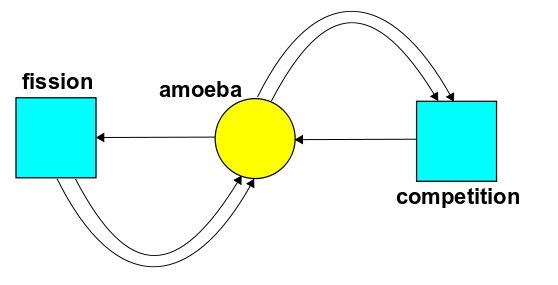It shows a world with one state, amoeba, and two transitions:

• reproduction, where one amoeba turns into two. Let's call the rate constant for this transition $\alpha$.
• competition, where two amoebas battle for resources and only one survives. Let's call the rate constant for this transition $\beta$.
• We are going to analyse this example in several ways. First we'll study the deterministic dynamics it describes: we'll look at its rate equation, which turns out to be the logistic equation, familiar in population biology. Then we'll study the stochastic dynamics, meaning its master equation. That's where the ideas from quantum field theory come in.

### The rate equation

If $P(t)$ is the population of amoebas at time $t$, we can follow the rules explained in Part 3 and crank out this rate equation: $$\frac{d P}{d t} = \alpha P - \beta P^2$$ We can rewrite this as $$\frac{d P }{d t}= k P(1-\frac{P}{Q})$$ where $$Q = \frac{\alpha}{\beta} , \qquad k = \alpha$$ What's the meaning of $Q$ and $k$?

• $Q$ is the carrying capacity, that is, the maximum sustainable population the environment can support.
• $k$ is the growth rate describing the approximately exponential growth of population when $P(t)$ is small.

It's a rare treat to find such an important differential equation that can be solved by analytical methods. Let's enjoy solving it.

We start by separating variables and integrating both sides: $$\int \frac{d P}{P (1-P/Q)} = \int k d t$$ We need to use partial fractions on the left side above, resulting in $$\int \frac{d P}{P} + \int \frac{d P}{Q-P} = \int k d t$$ and so we pick up a constant $C$, let $A=\pm e^{-C}$, and rearrange things as $$\frac{Q-P}{P}=A e^{-k t}$$ so the population as a function of time becomes $$P(t) = \frac{Q}{1+A e^{-k t}}$$ At $t=0$ we can determine $A$ uniquely. We write $P_0 := P(0)$ and $A$ becomes $$A = \frac{Q-P_0}{P_0}$$ The model now becomes very intuitive. Let's set $Q = k=1$ and make a plot for various values of $A$: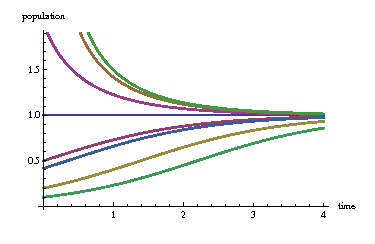We arrive at three distinct cases:

• equilibrium ($A=0$). The horizontal blue line corresponds to the case where the initial population $P_0$ exactly equals the carrying capacity. In this case the population is constant.
• dieoff ($A \lt 0$). The three decaying curves above the horizontal blue line correspond to cases where initial population is higher than the carrying capacity. The population dies off over time and approaches the carrying capacity.
• growth ($A \gt 0$). The four increasing curves below the horizontal blue line represent cases where the initial population is lower than the carrying capacity. Now the population grows over time and approaches the carrying capacity.

### The master equation

Next, let us follow the rules explained in Part 6 to write down the master equation for our example. Remember, now we write: $$\Psi(t) = \sum_{n = 0}^\infty \psi_n(t) z^n$$ where $\psi_n(t)$ is the probability of having $n$ amoebas at time $t$, and $z$ is a formal variable. The master equation says: $$\frac{d}{d t} \Psi(t) = H \Psi(t)$$ where $H$ is an operator on formal power series called the Hamiltonian. To get the Hamiltonian we take each transition in our Petri net and build an operator built from creation and annihilation operators, as follows. Reproduction works like this: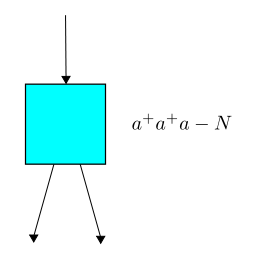while competition works like this: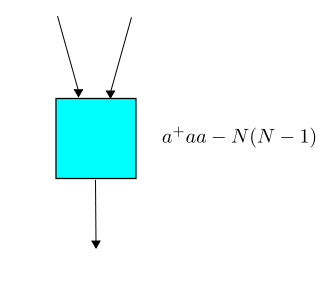Here $a$ is the annihilation operator, $a^\dagger$ is the creation operator and $N = a^\dagger a$ is the number operator. Last time John explained precisely how the $N$'s arise. So the theory is already in place, and we arrive at this Hamiltonian: $$H = \alpha (a^\dagger a^\dagger a - N) \;\; + \;\; \beta(a^\dagger a a - N(N-1))$$ Remember, $\alpha$ is the rate constant for reproduction, while $\beta$ is the rate constant for competition.

The master equation can be solved: it's equivalent to $\frac{d}{d t}(e^{-t H}\Psi(t))=0$ so that $e^{-t H}\Psi(t)$ is constant, and so $$\Psi(t) = e^{t H}\Psi(0)$$ and that's it! We can calculate the time evolution starting from any initial probability distribution of populations. Maybe everyone is already used to this, but I find it rather remarkable.

Here's how it works. We pick a population, say $n$ amoebas at $t=0$. This would mean $\Psi(0) = z^n$. We then evolve this state using $e^{t H}$. We expand this operator as $$\begin{array}{ccl} e^{t H} &=& \sum_{n=0}^\infty \frac{1}{n!} t^n H^n \\ \\ &=& 1 + t H + \frac{1}{2}t^2 H^2 + \cdots \end{array}$$ This operator contains the full information for the evolution of the system. It contains the histories of all possible amoeba populations—an amoeba mosaic if you will. From this, we can construct amoeba Feynman diagrams.

To do this, we work out each of the $H^n$ terms in the expansion above. The first-order terms correspond to the Hamiltonian acting once. These are proportional to either $\alpha$ or $\beta$. The second-order terms correspond to the Hamiltonian acting twice. These are proportional to either $\alpha^2$, $\alpha\beta$ or $\beta^2$. And so on.

This is where things start to get interesting! To illustrate how it works, we will consider two possibilities for the second-order terms:

1) We start with a lone amoeba, so $\Psi(0) = z$. It reproduces and splits into two. In the battle of the century, the resulting amoebas compete and one dies. At the end we have: $$\frac{\alpha \beta}{2} (a^\dagger a a)(a^\dagger a^\dagger a) z$$ We can draw this as a Feynman diagram: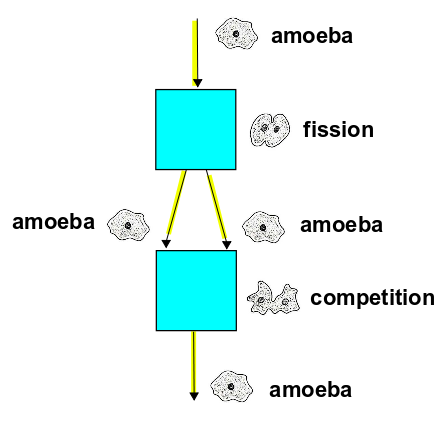You might find this tale grim, and you may not like the odds either. It's true, the odds could be better, but people are worse off than amoebas! The great Japanese swordsman Miyamoto Musashi quoted the survival odds of fair sword duels as 1/3, seeing that 1/3 of the time both participants die. A remedy is to cheat, but these amoeba are competing honestly.

2) We start with two amoebas, so the initial state is $\Psi(0) = z^2$. One of these amoebas splits into two. One of these then gets into an argument with the original amoeba over the Azimuth blog. The amoeba who solved all John's puzzles survives. At the end we have $$\frac{\alpha \beta}{2} (a^\dagger a a)(a^\dagger a^\dagger a) z^2$$ with corresponding Feynman diagram: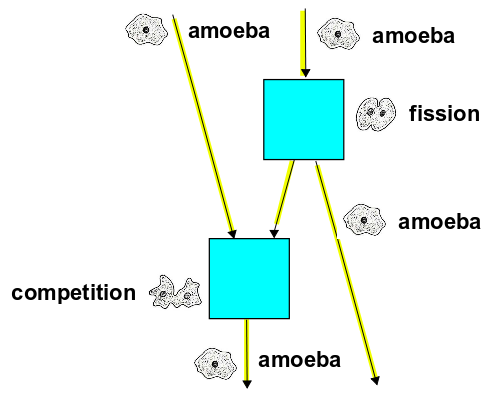This should give an idea of how this all works. The exponential of the Hamiltonian gives all possible histories, and each of these can be translated into a Feynman diagram. In a future blog entry, we might explain this theory in detail.

### An equilibrium state

We've seen the equilibrium solution for the rate equation; now let's look for equilibrium solutions of the master equation. This paper:

proves that for a large class of stochastic Petri nets, there exists an equilibrium solution of the master equation where the number of things in each state is distributed according to a Poisson distribution. Even more remarkably, these probability distributions are independent, so knowing how many things are in one state tells you nothing about how many are in another!

Here's a nice quote from this paper:

The surprising aspect of the deficiency zero theorem is that the assumptions of the theorem are completely related to the network of the system whereas the conclusions of the theorem are related to the dynamical properties of the system.

The 'deficiency zero theorem' is a result of Feinberg, which says that for a large class of stochastic Petri nets, the rate equation has a unique equilibrium solution. Anderson showed how to use this fact to get equilibrium solutions of the master equation!

We will consider this in future posts. For now, we need to talk a bit about 'coherent states'.

These are all over the place in quantum theory. Legend (or at least Wikipedia) has it that Erwin Schr�dinger himself discovered coherent states when he was looking for states of a quantum system that look 'as classical as possible'. Suppose you have a quantum harmonic oscillator. Then the uncertainty principle says that $$\Delta p \Delta q \ge \hbar/2$$ where $\Delta p$ is the uncertainty in the momentum and $\Delta q$ is the uncertainty in position. Suppose we want to make $\Delta p \Delta q$ as small as possible, and suppose we also want $\Delta p = \Delta q$. Then we need our particle to be in a 'coherent state'. That's the definition. For the quantum harmonic oscillator, there's a way to write quantum states as formal power series $$\Psi = \sum_{n = 0}^\infty \psi_n z^n$$ where $\psi_n$ is the amplitude for having $n$ quanta of energy. A coherent state then looks like this: $$\Psi = e^{c z} = \sum_{n = 0}^\infty \frac{c^n}{n!} z^n$$ where $c$ can be any complex number. Here we have omitted a constant factor necessary to normalize the state.

We can also use coherent states in classical stochastic systems like collections of amoebas! Now the coefficient of $z^n$ tells us the probability of having $n$ amoebas, so $c$ had better be real. And probabilities should sum to 1, so we really should normalize $\Psi$ as follows: $$\Psi = \frac{e^{c z}}{e^c} = e^{-c} \sum_{n = 0}^\infty \frac{c^n}{n!} z^n$$ Now, the probability distribution $$\psi_n = e^{-c} \; \frac{c^n}{n!}$$ is called a Poisson distribution. So, for starters you can think of a 'coherent state' as an over-educated way of talking about a Poisson distribution.

Let's work out the expected number of amoebas in this Poisson distribution. In the answers to the puzzles in Part 6, we started using this abbreviation: $$\sum \Psi = \sum_{n = 0}^\infty \psi_n$$ We also saw that the expected number of amoebas in the probability distribution $\Psi$ is $$\sum N \Psi$$ What does this equal? Remember that $N = a^\dagger a$. The annihilation operator $a$ is just $\frac{d}{d z}$, so $$a \Psi = c \Psi$$ and we get $$\sum N \Psi = \sum a^\dagger a \Psi = c \sum a^\dagger \Psi$$ But we saw in Part 5 that $a^\dagger$ is stochastic, meaning $$\sum a^\dagger \Psi = \sum \Psi$$ for any $\Psi$. Furthermore, our $\Psi$ here has $$\sum \Psi = 1$$ since it's a probability distribution. So: $$\sum N \Psi = c \sum a^\dagger \Psi = c \sum \Psi = c$$ The expected number of amoebas is just $c$.

Puzzle 1. This calculation must be wrong if $c$ is negative: there can't be a negative number of amoebas. What goes wrong then?

Puzzle 2. Use the same tricks to calculate the standard deviation of the number of amoebas in the Poisson distribution $\Psi$.

Now let's return to our problem and consider the initial amoeba state $$\Psi = e^{c z}$$ Here aren't bothering to normalize it, because we're going to look for equilibrium solutions to the master equation, meaning solutions where $\Psi(t)$ doesn't change with time. So, we want to solve $$H \Psi = 0$$ Since this equation is linear, the normalization of $\Psi$ doesn't really matter.

Remember, $$H\Psi = \alpha (a^\dagger a^\dagger a - N)\Psi + \beta(a^\dagger a a - N(N-1)) \Psi$$ Let's work this out. First consider the two $\alpha$ terms: $$a^\dagger a^\dagger a \Psi = c z^2 \Psi$$ and $$-N \Psi = -a^\dagger a\Psi = -c z \Psi$$ Likewise for the $\beta$ terms we find $$a^\dagger a a\Psi=c^2 z \Psi$$ and $$-N(N-1)\psi = -a^\dagger a^\dagger a a \Psi = -c^2 z^2\Psi$$ Here I'm using something John showed in Part 6: the product ${a^\dagger}^2 a^2$ equals the 'falling power' $N(N-1)$.

The sum of all four terms must vanish. This happens whenever $$\alpha(c z^2 - c z)+\beta(c^2 z-c^2 z^2) = 0$$ which is satisfied for $$c= \frac{\alpha}{\beta}$$ Yipee! We've found an equilibrium solution, since we found a value for $c$ that makes $H \Psi = 0$. Even better, we've seen that the expected number of amoebas in this equilibrium state is $$\frac{\alpha}{\beta}$$ This is just the same as the equilibrium population we saw in the rate equation—that is, the logistic equation! That's pretty cool, but it's no coincidence: in fact, Anderson proved it works like this for lots of stochastic Petri nets.

I'm not sure what's up next or what's in store, since I'm blogging at gun point from inside a rabbit cage:I'd imagine we're going to work out the theory behind this example and prove the existence of equilibrium solutions for master equations more generally. One idea John had was to have me start a night shift—that way you'll get Azimuth posts 24 hours a day.

Puzzle 1. We calculated that the expected number of amoebas in the Poisson distribution with parameter $c$ is equal to $c$. But this can't be true if $c$ is negative: there can't be a negative number of amoebas. What goes wrong then?
Answer. If the probability of having $n$ amoebas is given by the Poisson distribution $$\psi_n = e^{-c} \; \frac{c^n}{n!}$$ then $c$ had better be nonnegative for the probability to be negative when $c = 1$.
Answer. The standard deviation is the square root of the variance, which is $$\sum N^2 \Psi - (\sum N \Psi)^2$$ We have seen that for the Poisson distribution, $$\sum N \Psi = c$$ and using the same tricks we see $$\begin{array}{ccl} \sum N^2 \Psi &=& \sum a^{\dagger} a a^{\dagger} a \Psi \\ &=& c \sum a^{\dagger} a a^{\dagger} \Psi \\ &=& c \sum a a^{\dagger} \Psi \\ &=& c \sum (a^{\dagger} a + 1) \Psi \\ &=& c(c + 1)\\ \end{array}$$ So, the variance is $c(c+1) - c^2 = c$ and the standard deviation is $\sqrt{c}$.